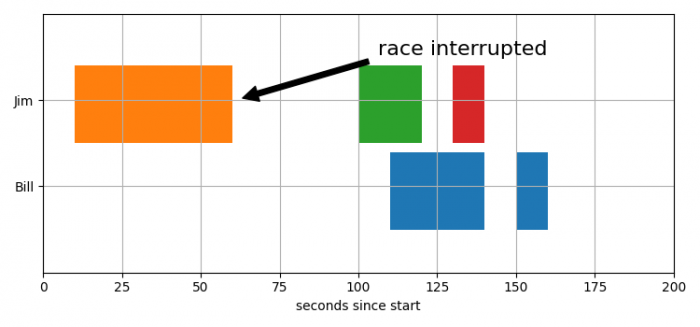# How to make a broken horizontal bar plot in Matplotlib?

We can take the following steps to make a broken bar plot,

• Set the figure size and adjust the padding between and around the subplots.
• Create a figure and a set of subplots.
• Plot a horizontal sequence of rectangles.
• Set x and y axes scale, X-axis label, Y ticks and Y tick labels.
• Configure the grid lines.
• Use annotate() method to show text that can refer to a specific position.
• To display the figure, use show() method.

## Example

import matplotlib.pyplot as plt

plt.rcParams["figure.figsize"] = [7.50, 3.50]
plt.rcParams["figure.autolayout"] = True

fig, ax = plt.subplots()

ax.broken_barh([(110, 30), (150, 10)], (10, 9), facecolors='tab:blue')
ax.broken_barh([(10, 50), (100, 20), (130, 10)], (20, 9),
facecolors=('tab:orange', 'tab:green', 'tab:red'))
ax.set_ylim(5, 35)
ax.set_xlim(0, 200)
ax.set_xlabel('seconds since start')
ax.set_yticks([15, 25])
ax.set_yticklabels(['Bill', 'Jim'])
ax.grid(True)

ax.annotate('race interrupted', (61, 25),
xytext=(0.8, 0.9), textcoords='axes fraction',
arrowprops=dict(facecolor='black', shrink=0.05),
fontsize=16,
horizontalalignment='right', verticalalignment='top')

plt.show()

## Output